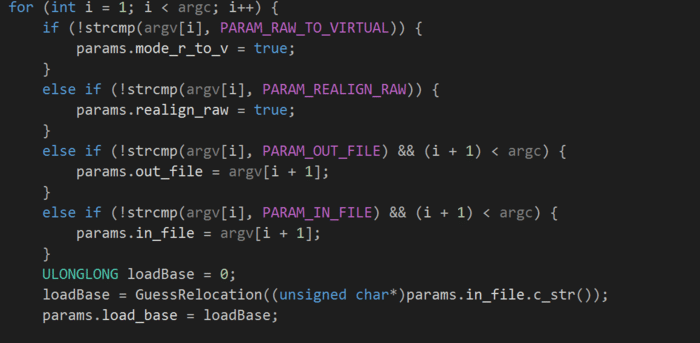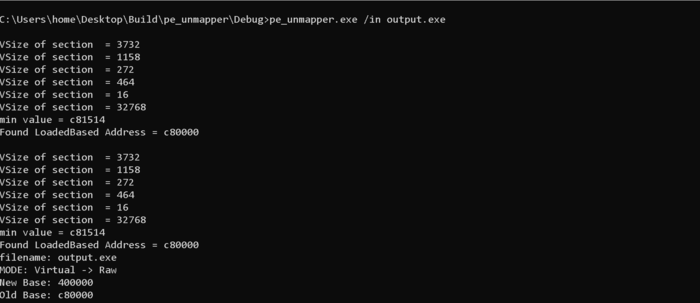# Relocating BaseAddress Agnostic Memory Dumps

Often times we need a loaded base address of a memory image that needs to be disk realigned in order to load it and parse the binary successfully in binary analysis tools like IDA or debuggers .

During linking phase the `Preferred Base Address` is selected and all absolute addresses are set relative to this particular address .

Relocation table for a PE file consists of following fields

``````typedef struct _IMAGE_BASE_RELOCATION {
DWORD   SizeOfBlock;
//  WORD    TypeOffset;
} IMAGE_BASE_RELOCATION;
``````

The `VirtualAddress` consists virtual offset to a 4kb page where relocations are applied to that page , `sizeofblock` is size of 2 byte array consists of offsets to relocation targets in the same page . Base relocation can have multiple relocation entries .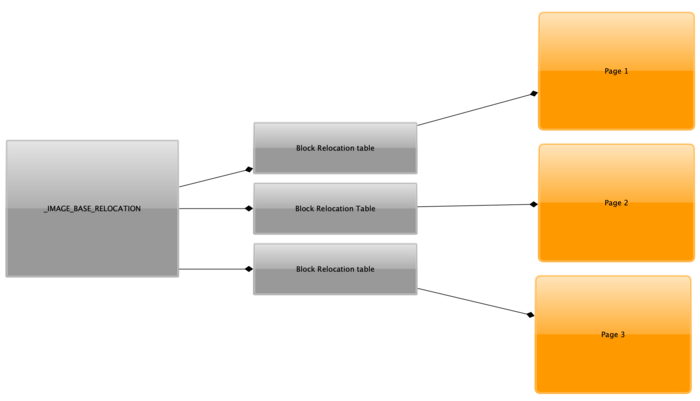During the image loading process , if the `LoadedBase` address is different from preferred base address ( most cases, if ASLR is enabled ) , binary relocation must be done . Following steps are needed to take place to successfully relocate an image loaded in memory .

• Retrieve the new loaded image base

• Calculate the `Relocation Delta  `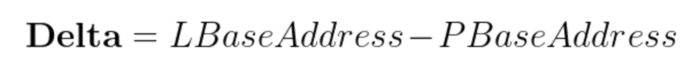And thus the binary is relocated, as per the new image address with proper fix-ups processed
And the single most important ingredient required was the `Loaded image base` .

But there is a catch in all of this ! Let us suppose that we are given a memory dump , but without a known `LoadedBase` address . In that case the conundrum is to relocate the base image but without a delta

Can we correctly predict the base address in this kind of scenario?

Actually , we can! The information presented by `_IMAGE_BASE_RELOCATION` and multiple values at page offsets do allow us for some kind of side channel attack to predict the `LoadedBase` address correctly

The way to do this is to first determine the `offset` or `RVA``of a``value at fixup``from an offset and a``fixup`` where the page difference is less than 4KB ( Page Size )

This diagram illustrates this idea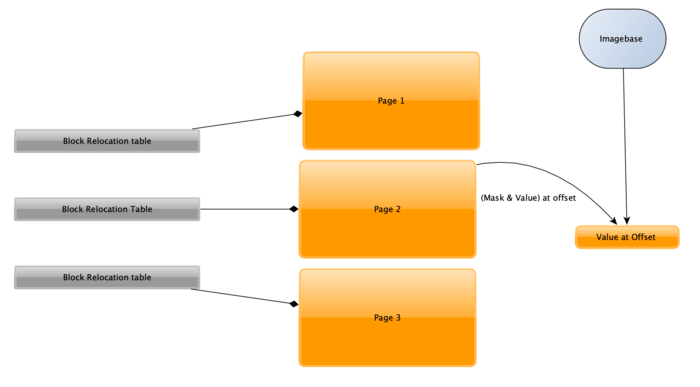It involves the following steps

• Enumerate all values at `fix-ups` and select the smallest of it ( nearest to base address )
• Enumerate all relocation table RVA’s and calculate a mask to strip the bits equal to the bits of RVA
• Subtract the two values , and if the difference is less than 4096 ( Page size) the value of offset is probably present in the same page .
• Calculate the distance from base by subtracting `fixup` , `offset from page base` , and `RVA` from the relocation base all together.

This all can be implemented by a python script , script given below takes a memory dump image ( file system aligned ) without the loaded base and determines the base address and performs the `fixups`

``````import pefile

import struct
import sys
import argparse

def Realign():
parser = argparse.ArgumentParser(description='Image Relocater ( without the known Imagebase ) (C) Raashid Bhat')
parser.add_argument('outfile', type=str, help='Output relocated and realigned file')
args = parser.parse_args()

pe =pefile.PE(data =PeFile)
Size = 0
for section in pe.sections:
break
for section in pe.sections:

print "Size = %d" % Size
FinData = bytearray("\x00" * Size, "utf-8")
FirstSec = 1
for section in pe.sections:
if FirstSec:
FinData[0: section.PointerToRawData] = PeFile[BaseAdd : section.PointerToRawData]
FirstSec = 0
continue

fixups = []
rva = []
for i in relocData:

for j in i.entries:
if j.type != 3:
continue

FixUp = struct.unpack("<I", str(FinData[j.rva : j.rva + 4]) )

fixups.append(FixUp)

while pRelocEntry:

rva.append(pRelocEntry)
print "RVA %x size = %d"  % (pRelocEntry, SizeOfBlock)

print "Minumum Value at offset %s " % hex(min(fixups))
for i in rva:
Mask = int("f" * len(hex(i)[2:] ), 16)

OffsetRVA = (min(fixups) & Mask) - i

if OffsetRVA < 4096 and OffsetRVA >= 0 : # page size 4096 KB
print "Found Loaded Base Address 0x%x" % (min(fixups) - OffsetRVA - i)
delta  = (min(fixups) - OffsetRVA - i) -  pe.OPTIONAL_HEADER.ImageBase

for i in relocData:
for j in i.entries:
if j.type != 3:
continue

FixUp = struct.unpack("<I", str(FinData[j.rva : j.rva + 4]) ) - delta

fixups.append(FixUp)

FinData[j.rva :j.rva + 4] = struct.pack("I", FixUp)
#print j.type

Unexec = ""
FirstSec = 1
for section in pe.sections:
if FirstSec:
UnExec = (FinData[0: section.PointerToRawData])
FirstSec = 0
continue

open(args.outfile, "wb").write(UnExec)
return FinData

if __name__ == '__main__':
Realign()

``````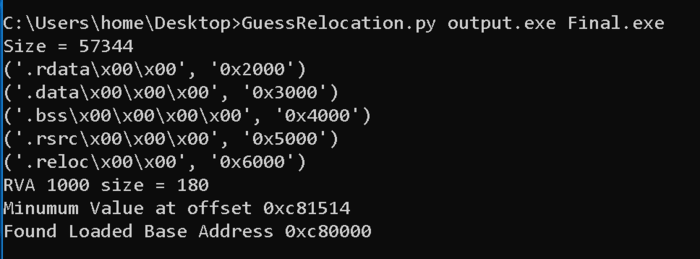There is a cool project known as `pe_unmapper`(https://github.com/hasherezade/libpeconv/tree/master/pe_unmapper) by hasherezade which helps in unexecing the memory dump into raw images . It also performs the necessary relocations . I implemented the above mentioned technique in pe_unmapper to relocate binaries without a need of `loadedbase` address . pe_unmapper takes the following arguments , one of them being `loadedbase` , but with the necessary modifications it works without `loadedbase` being provided .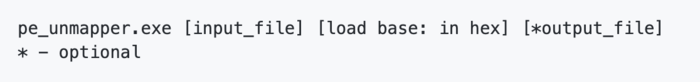``````#define WIN32_LEAN_AND_MEAN
#include <windows.h>

#include <stdio.h>
#include <winnt.h>
#include <vector>
#include <iostream>
#include <algorithm>
#include <string>

using namespace std;

unsigned char ImageName[0x100];

int countDigit(unsigned int n)
{
if (n == 0)
return 0;
return 1 + countDigit(n / 10);
}

unsigned int GuessRelocation(unsigned char *fileName)
{
unsigned short iNumSec = 0;

vector<unsigned int> ValAtFixups;
vector<unsigned int> RVA;
FILE *fp = NULL;
PIMAGE_BASE_RELOCATION RelocTable = NULL;

IMAGE_DOS_HEADER DosHdr = { 0 };
IMAGE_FILE_HEADER FileHdr = { 0 };
IMAGE_OPTIONAL_HEADER OptHdr = { 0 };
PIMAGE_BASE_RELOCATION pRelocEntry = NULL;
unsigned int RelocBlockSize = 0;
unsigned int *FixUp = 0;
unsigned char *pMappedImage = NULL;

int i = 0;
unsigned int MZ_PE_LEN = 0;
unsigned short  iHdrLen = 0;
unsigned int delta = 0;

fp = fopen((const char*)fileName, "rb");

fseek(fp, (unsigned int)DosHdr.e_lfanew + 4, SEEK_SET);

while (iNumSec < FileHdr.NumberOfSections)
{
iNumSec++;
}

MZ_PE_LEN = ftell(fp);
iHdrLen = MZ_PE_LEN;

while (i < iNumSec)
{

printf("\nVSize of section  = %d", pTail[i].Misc.VirtualSize);

i++;
}
i = 0;
pMappedImage = (unsigned char*)VirtualAlloc(0, sizeof(char)  * MZ_PE_LEN + 10, MEM_COMMIT, PAGE_EXECUTE_READWRITE);

fseek(fp, 0, SEEK_SET);

i = 0;
while (i < iNumSec)
{
fseek(fp, pTail[i].PointerToRawData, SEEK_SET);

i++;
}

{

RelocBlockSize = (pRelocEntry->SizeOfBlock - 8) / 2;
pRelocEntry = (PIMAGE_BASE_RELOCATION) (unsigned char*)pRelocEntry + 8;

while (RelocBlockSize--)
{
if (*(unsigned short*)pRelocEntry == 0x3000)
{
pRelocEntry = (PIMAGE_BASE_RELOCATION)(unsigned char*)pRelocEntry + 2;
continue;
}
FixUp = (unsigned int*)(*(unsigned short*)pRelocEntry & 0x0fff);

RVA.insert(RVA.begin(), (unsigned int )FixUp + (unsigned int )iRelocVaddr);

FixUp = (unsigned int*)((unsigned int)FixUp + ((unsigned int)pMappedImage + (unsigned int)iRelocVaddr));

ValAtFixups.insert(ValAtFixups.begin(), *FixUp);
pRelocEntry = (PIMAGE_BASE_RELOCATION) ((unsigned char*)pRelocEntry + 2);

}

}
printf("\nmin value = %x", *min_element(ValAtFixups.begin(), ValAtFixups.end()));
unsigned int minValOffset = *min_element(ValAtFixups.begin(), ValAtFixups.end());

vector<unsigned int>::iterator it;
for (it = RVA.begin(); it != RVA.end(); it++) {

int i = 0;
string str;
char digit = {0};
itoa(*it, digit, 10);
for (; i < strlen(digit); i++)
str.push_back('f');

unsigned int Mask = stoi(str, 0, 16);

unsigned int OffsetRVA = (minValOffset & Mask) - *it;

if (OffsetRVA < 4096 && OffsetRVA >= 0)
{
return minValOffset - OffsetRVA - *it;
}

}

fclose(fp);
return 0;
}
``````